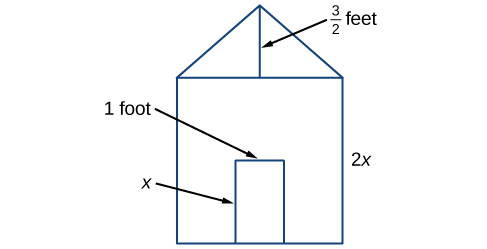## Introduction to Polynomials

### Learning Objectives

By the end of this section, you will be able to:

• Identify the degree and leading coefficient of polynomials.
• Multiply polynomials.
• Use FOIL to multiply binomials.
• Perform operations with polynomials of several variables.

Earl is building a doghouse, whose front is in the shape of a square topped with a triangle. There will be a rectangular door through which the dog can enter and exit the house. Earl wants to find the area of the front of the doghouse so that he can purchase the correct amount of paint. Using the measurements of the front of the house, shown in Figure 1, we can create an expression that combines several variable terms, allowing us to solve this problem and others like it.Figure 1

First find the area of the square in square feet.

$\begin{array}{ccc}\hfill A& =& {s}^{2}\hfill \\ & =& {\left(2x\right)}^{2}\hfill \\ & =& 4{x}^{2}\hfill \end{array}$

Then find the area of the triangle in square feet.

$\begin{array}{ccc}\hfill A& =& \frac{1}{2}bh\hfill \\ & =& \text{ }\frac{1}{2}\left(2x\right)\left(\frac{3}{2}\right)\hfill \\ & =& \text{ }\frac{3}{2}x\hfill \end{array}$

Next find the area of the rectangular door in square feet.

$\begin{array}{ccc}\hfill A& =& lw\hfill \\ & =& x\cdot 1\hfill \\ \hfill & =& x\hfill \end{array}$

The area of the front of the doghouse can be found by adding the areas of the square and the triangle, and then subtracting the area of the rectangle. When we do this, we get $4{x}^{2}+\frac{3}{2}x-x{\text{ft}}^{2}$, or $4{x}^{2}+\frac{1}{2}x$ ft2.

In this section, we will examine expressions such as this one, which combine several variable terms.